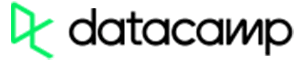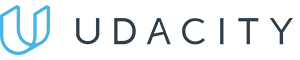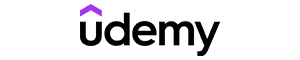Code has been added to clipboard!

# Learn to PHP Sort Array by Key or Value: Tips and Examples

Published Aug 8, 2017
Updated Oct 2, 2019

PHP arrays are useful when developers store data in variables. You can group them by creating specific categories and placing all related values into lists.

Sometimes arrays may contain too many values, and their management becomes complicated. To simplify the manipulation of arrays, PHP introduces PHP sort array functions that are supposed to help you organize these lists.

## PHP Sort Array: Main Tips

• PHP offers multiple built-in functions for PHP array sorting.
• Some of the functions can only be used for associative arrays.
• It's possible to array sort PHP by key or by value, in numerical, alphabetical, descending and ascending orders.

## Function Types for Sorting

Let's look at the various PHP array sorting functions. First, we have the `sort()` method used to array sort PHP code in an ascending order. For a descending order, use `rsort`.

There are four functions for associative arrays — you either array sort PHP by key or by value.

To PHP sort array by key, you should use `ksort()` (for ascending order) or `krsort()` (for descending order). To PHP sort array by value, you will need functions `asort()` and `arsort()` (for ascending and descending orders).

### sort()

`sort()` function sorts an array in an ascending order.

Let's look at an example with a PHP array that holds names of different guitar manufacturers. The code reveals how this function sorts the array in alphabetical order:

Example
``````<?php
\$guitars = ['Warvick', 'Gibson', 'Fender'];
sort(\$guitars);
?>``````

Here's another example. This time the array holds numbers and sorts them in numerical order:

Example
``````<?php
\$numerals = [5, 7, 3, 23, 12];
sort(\$numerals);
?>``````Pros
• Easy to use with a learn-by-doing approach
• Offers quality content
• Gamified in-browser coding experience
• The price matches the quality
• Suitable for learners ranging from beginner to advanced
Main Features
• Free certificates of completion
• Focused on data science skills
• Flexible learning timetablePros
• Simplistic design (no unnecessary information)
• High-quality courses (even the free ones)
• Variety of features
Main Features
• Nanodegree programs
• Suitable for enterprises
• Paid Certificates of completionPros
• Easy to navigate
• No technical issues
• Seems to care about its users
Main Features
• Huge variety of courses
• 30-day refund policy
• Free certificates of completion

### rsort()

`rsort()` sorts the array in a descending order.

Let's use it in the same script we saw in the example with guitar companies. The change of function will produce a different result:

Example
``````<?php
\$guitars = ['Fender', 'Gibson', 'Warvick'];
rsort(\$guitars);
?>``````

Let's do that with numbers. You will notice the script produces an opposite result than `sort()` did in the previous example:

Example
``````<?php
\$numerals = [5, 7, 3, 23, 12];
rsort(\$numerals);
?>``````

## asort() and arsort(): Sorting by Value

`asort()` and `arsort()` are used to PHP sort associative arrays by their value.

For demonstration, we will use simple examples where the values refer to guys' weights. Since the values here are numerical, the array will be sorted in that order.

We use `asort()` for the ascending order:

Example
``````<?php
\$weight = [
'Pete' => 75,
'Benjamin' => 62,
'Jonathan' => 101
];
asort(\$weight);
?>``````

When we need our array sorted by key in an descending order, we choose `arsort()`:

Example
``````<?php
\$weight = [
'Pete' => 75,
'Benjamin' => 89,
'Jonathan' => 101
];
arsort(\$weight);
?>``````

## ksort() and krsort(): Sorting by Key

`ksort()` and `krsort()` make PHP sort associative arrays, but they don't do it by value: what matters here is the key. In our example, names were the keys. Hence, using these two functions will sort out guys not by weight but by their names (alphabetically).

We can use `ksort()` for an ascending order:

Example
``````<?php
\$weight = [
'Pete' => 75,
'Benjamin' => 89,
'Jonathan' => 101
];
ksort(\$weight);
?>``````

If descending order sounds more acceptable, we choose `krsort()`:

Example
``````<?php
\$weight = [
'Pete' => 75,
'Benjamin' => 89,
'Jonathan' => 101
];
krsort(\$weight);
?>``````

## PHP Sort Array: Summary

• You can easily sort PHP arrays using PHP inbuilt functions.
• It's possible to PHP sort array by key or by value, in numerical, alphabetical, descending and ascending orders.
• Some functions can only be applied for associative arrays.

Days
Hours
Minutes
Seconds